Next: Continuous Eigenvalues Up: Fundamentals of Quantum Mechanics Previous: Eigenstates and Eigenvalues

# Measurement

Suppose thatis an Hermitian operator corresponding to some dynamical variable. By analogy with the discussion in Sect. 3.16, we expect that if a measurement ofyields the resultthen the act of measurement will cause the wavefunction to collapse to a state in which a measurement ofis bound to give the result. What sort of wavefunction,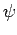, is such that a measurement ofis bound to yield a certain result,? Well, expressingas a linear combination of the eigenstates of, we have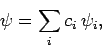(266)

whereis an eigenstate ofcorresponding to the eigenvalue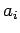. If a measurement ofis bound to yield the resultthen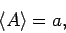(267)

and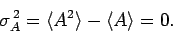(268)

Now it is easily seen that(269)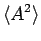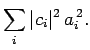(270)

Thus, Eq. (268) gives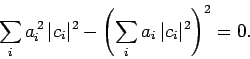(271)

Furthermore, the normalization condition yields(272)

For instance, suppose that there are only two eigenstates. The above two equations then reduce to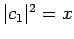, and, where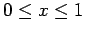, and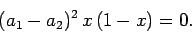(273)

The only solutions areand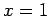. This result can easily be generalized to the case where there are more than two eigenstates. It follows that a state associated with a definite value ofis one in which one of the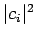is unity, and all of the others are zero. In other words, the only states associated with definite values ofare the eigenstates of. It immediately follows that the result of a measurement ofmust be one of the eigenvalues of. Moreover, if a general wavefunction is expanded as a linear combination of the eigenstates of, like in Eq. (266), then it is clear from Eq. (269), and the general definition of a mean, that the probability of a measurement ofyielding the eigenvalueis simply, where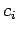is the coefficient in front of theth eigenstate in the expansion. Note, from Eq. (272), that these probabilities are properly normalized: i.e., the probability of a measurement ofresulting in any possible answer is unity. Finally, if a measurement ofresults in the eigenvaluethen immediately after the measurement the system will be left in the eigenstate corresponding to.

Consider two physical dynamical variables represented by the two Hermitian operatorsand. Under what circumstances is it possible to simultaneously measure these two variables (exactly)? Well, the possible results of measurements ofandare the eigenvalues ofand, respectively. Thus, to simultaneously measureand(exactly) there must exist states which are simultaneous eigenstates ofand. In fact, in order forandto be simultaneously measurable under all circumstances, we need all of the eigenstates ofto also be eigenstates of, and vice versa, so that all states associated with unique values ofare also associated with unique values of, and vice versa.

Now, we have already seen, in Sect. 4.8, that ifanddo not commute (i.e., if) then they cannot be simultaneously measured. This suggests that the condition for simultaneous measurement is thatandshould commute. Suppose that this is the case, and that theandare the normalized eigenstates and eigenvalues of, respectively. It follows that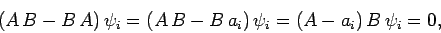(274)

or(275)

Thus,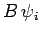is an eigenstate ofcorresponding to the eigenvalue(though not necessarily a normalized one). In other words,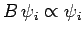, or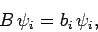(276)

where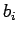is a constant of proportionality. Hence,is an eigenstate of, and, thus, a simultaneous eigenstate ofand. We conclude that ifandcommute then they possess simultaneous eigenstates, and are thus simultaneously measurable (exactly).Next: Continuous Eigenvalues Up: Fundamentals of Quantum Mechanics Previous: Eigenstates and Eigenvalues
Richard Fitzpatrick 2010-07-20